# Properties of Matter Properties of Matter Kinetic Theory

• Slides: 18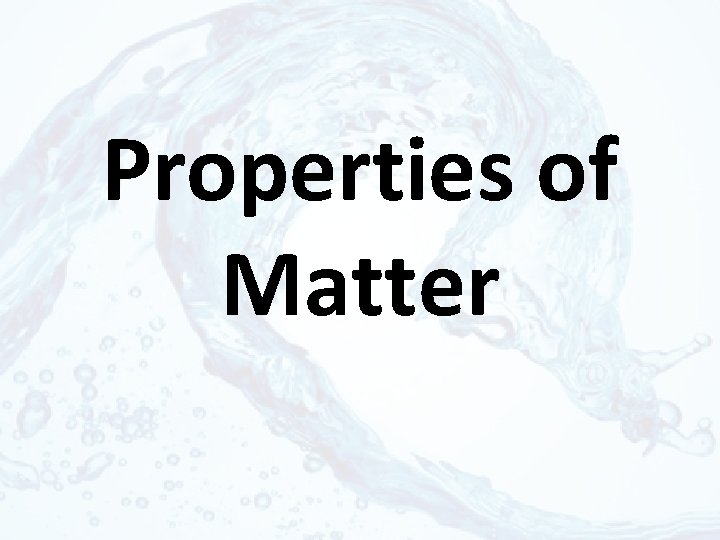Properties of Matter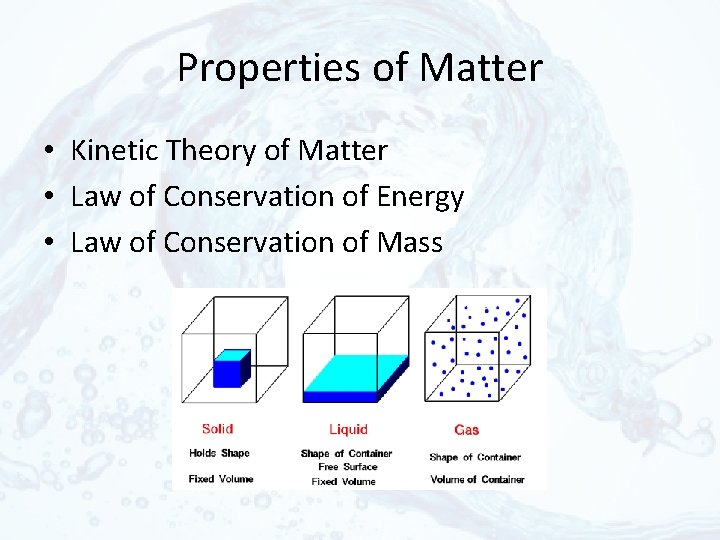Properties of Matter • Kinetic Theory of Matter • Law of Conservation of Energy • Law of Conservation of MassKinetic Theory of Matter • All matter is made of particles called atoms. • These atoms are always in constant motion. • As heat and energy increase, motion of the atoms increases.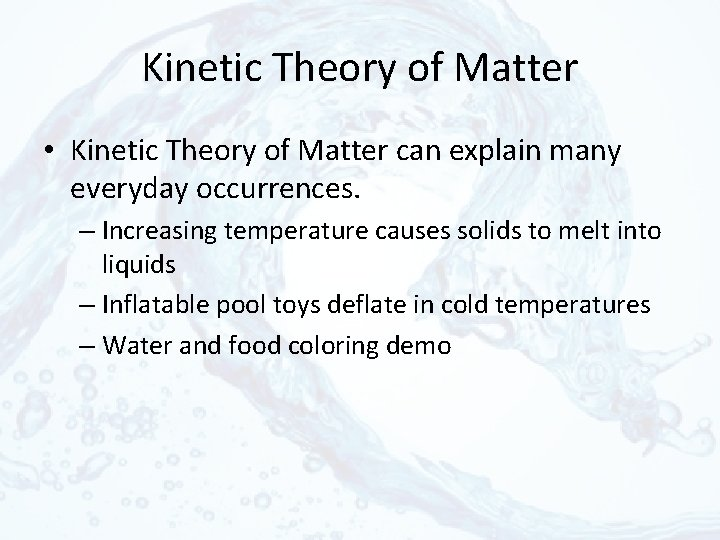Kinetic Theory of Matter • Kinetic Theory of Matter can explain many everyday occurrences. – Increasing temperature causes solids to melt into liquids – Inflatable pool toys deflate in cold temperatures – Water and food coloring demo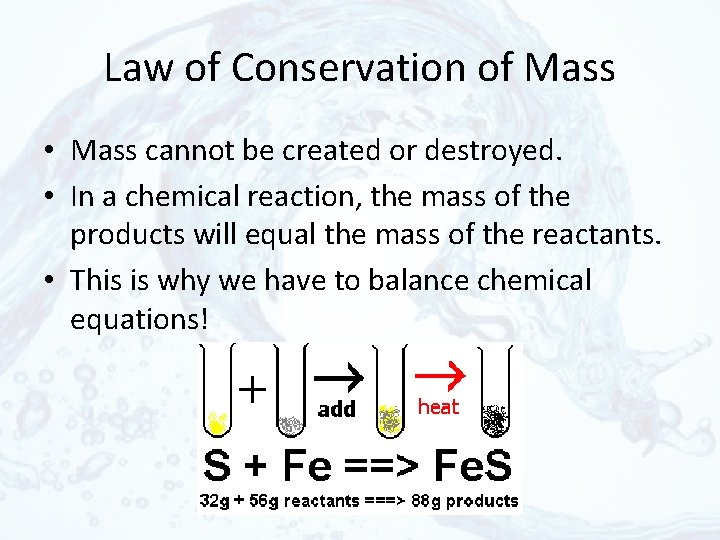Law of Conservation of Mass • Mass cannot be created or destroyed. • In a chemical reaction, the mass of the products will equal the mass of the reactants. • This is why we have to balance chemical equations!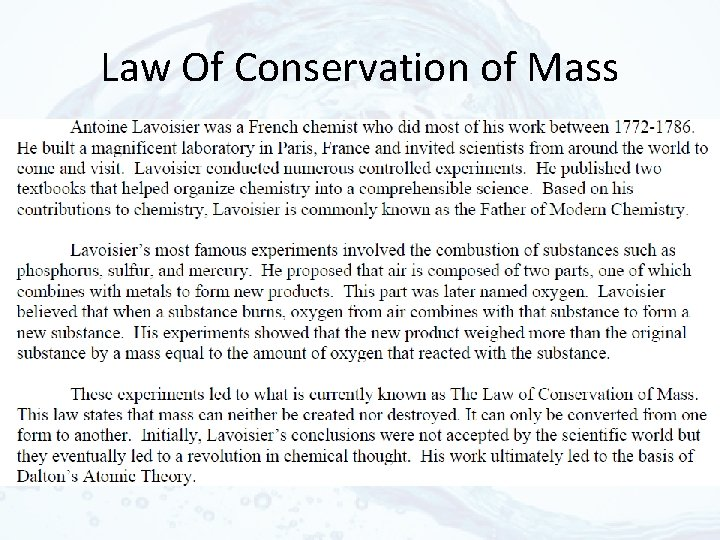Law Of Conservation of MassLaw of Conservation of Mass • You place 10 grams of ice in a beaker and let it melt. What is the mass of the liquid in the beaker? • Baking soda and vinegar experiment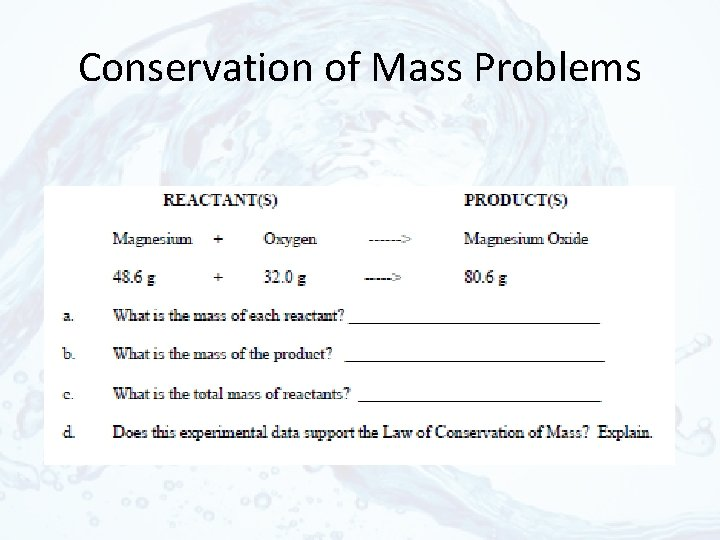Conservation of Mass Problems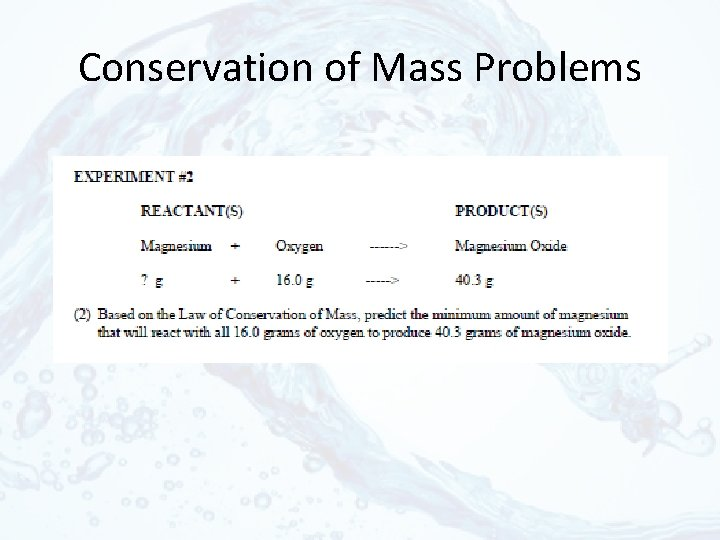Conservation of Mass ProblemsConservation of Mass ProblemsConservation of Mass ProblemsLaw of Conservation of Energy • Energy cannot be created or destroyed • Energy can change forms – Heat – Electrical – Chemical – Mechanical – Any more? ?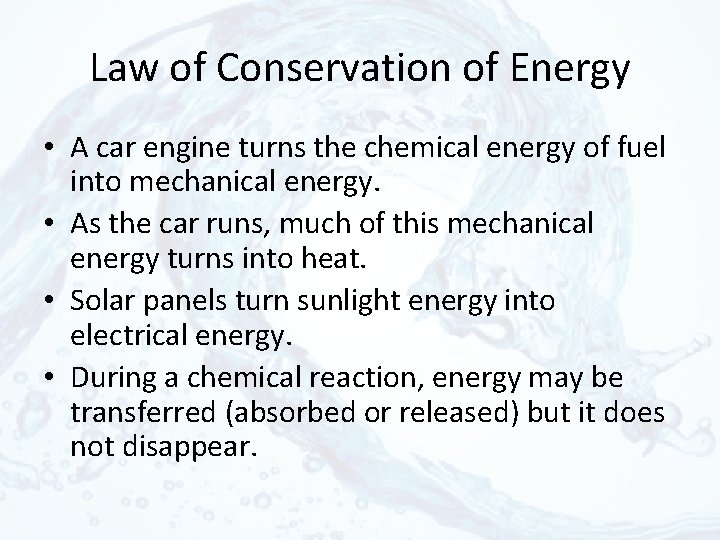Law of Conservation of Energy • A car engine turns the chemical energy of fuel into mechanical energy. • As the car runs, much of this mechanical energy turns into heat. • Solar panels turn sunlight energy into electrical energy. • During a chemical reaction, energy may be transferred (absorbed or released) but it does not disappear.Energy Questions 1. What is the definition of energy? 2. Describe and give examples of two types of kinetic energy. 3. Describe and give examples of two types of potential energy. 4. Give an example of an energy transformation. 5. What does energy efficiency refer to? 6. Do you think a car engine is energy efficient? Why or why not? 7. If energy cannot be created or destroyed, why are we worried about running out of energy sources?Foldable/Poster Guidelines • For each property of matter: – Define it – Draw it – Apply it (where can you see this in real life? )